# Basic Voltmeter Use

## Basic Electricity

• #### Question 1

What will this voltmeter register when connected to a battery as shown (assume a battery voltage of 6 volts)? Explain your answer.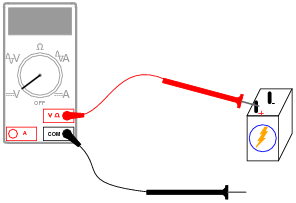• #### Question 2

What will this voltmeter register when connected to a battery as shown (assume a battery voltage of 6 volts)? Explain your answer.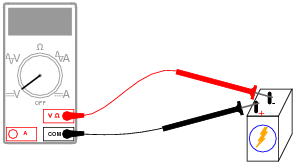• #### Question 3

If we were to connect a voltmeter directly to an ohmmeter, what would you expect to see the ohmmeter register, for resistance between its test leads?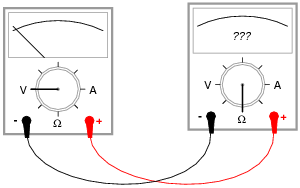• #### Question 4

Many electronic circuits use what is called a split or a dual power supply:Determine what a digital voltmeter would indicate if connected between the following points:

NOTE: in electronic systems, “ground” is often not associated with an actual earth-soil contact. It usually only refers to a common point of reference somewhere in the circuit used to take voltage measurements. This allows us to specify voltages at single points in the circuit, with the implication that “ground” is the

other point for the voltmeter to connect to.

• #### Question 5

Determine how much voltage a voltmeter would indicate when connected between the following points in this circuit:• Between TB1-1 and TB1-3
• Between TB1-4 and TB2-4
• Between TB2-3 and TB2-1
• Between TB1-1 and TB2-1

Hint: it might help to draw a neat schematic diagram of this circuit first, with all connection points labeled!

• #### Question 6

Determine how much voltage a voltmeter would indicate when connected between the following points in this faulted circuit:• Between TB1-1 and TB1-3
• Between TB1-4 and TB2-4
• Between TB2-3 and TB2-1
• Between TB1-1 and TB2-1

Hint: it might help to draw a neat schematic diagram of this circuit first, with all connection points labeled!

• #### Question 7

Determine what these four voltmeters (A, B, C, D) will register when connected to this circuit in the following positions (assume a battery voltage of 6 volts):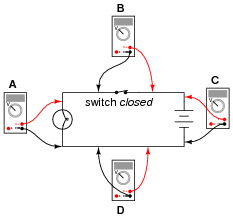• Voltmeter A =
• Voltmeter B =
• Voltmeter C =
• Voltmeter D =
• #### Question 8

Determine what these four voltmeters (A, B, C, D) will register when connected to this circuit in the following positions (assume a battery voltage of 6 volts):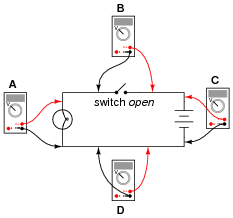• Voltmeter A =
• Voltmeter B =
• Voltmeter C =
• Voltmeter D =
• #### Question 9

Shown here is a circuit constructed on a PCB (a “Printed Circuit Board”), with copper “traces” serving as wires to connect the components together: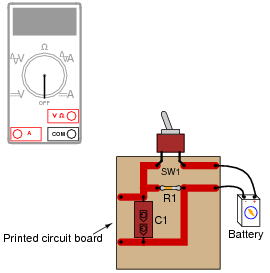How would the multimeter be used to measure the voltage across the component labeled “R1” when energized? Include these important points in your answer:

• The configuration of the multimeter (selector switch position, test lead jacks)
• The connections of the meter test leads to the circuit
• The state of the switch on the PCB (open or closed)
• #### Question 10

Suppose I were about to measure an unknown voltage with a manual-range voltmeter. This particular voltmeter has several different voltage measurement ranges to choose from:

• 500 volts
• 250 volts
• 100 volts
• 50 volts
• 25 volts
• 10 volts
• 5 volts

What range would be best to begin with, when first measuring this unknown voltage with the meter? Explain your answer.

• #### Question 11

Suppose I needed to test for the presence of DC voltage between all wires connected to this terminal block. What is the fewest number of individual measurements I would have to perform with with a voltmeter in order to test for voltage between all possible wire pair combinations (remember that voltage is always measured between two points!)?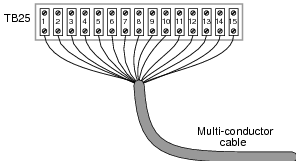• #### Question 12

Voltmeters must be connected in parallel with the voltage to be measured:In order to practically function, an voltmeter must have some finite amount of internal resistance. It is usually a very large amount, but less than infinite. It should be apparent to you that the presence of this resistance will have some effect on the circuit voltage, when compared to the amount of voltage in the circuit without any meter connected, due to the fact that real voltage sources tend to “sag” when subjected to additional loads:Explain why it is usually safe to ignore the internal resistance of an voltmeter, though, when it is in a circuit. A common term used in electrical engineering to describe this intentional oversight is swamping. In this particular circuit an engineer would say, “The resistance of the light bulb swamps the internal resistance of the voltmeter.”